Thursday, April 14, 2011

Manifolds: Curvature and Construction II

This post is part of the Manifolds series. For the previous post in the series, see Manifolds: Curvature and Construction.

In the previous post, fundamental polygons were introduced. A further exploration of these figures is important in constructing manifolds. However, we find that there are limits to the accuracy to which even two-dimensional manifolds can be represented in three-dimensional space. Take the torus for example. It was found to have a fundamental polygon ABA*B*. All four corners of the four-sided fundamental polygon eventually coincide when the torus is constructed. As a result, the angular measure around the resulting point on the manifold is exactly 360º, and the manifold is Euclidean at that point.

This result can be expanded by considering the symmetry of the torus. In fact, any point can be chosen as the coincidence of the four corners. This means that the geometry around every point on a torus is perfectly flat.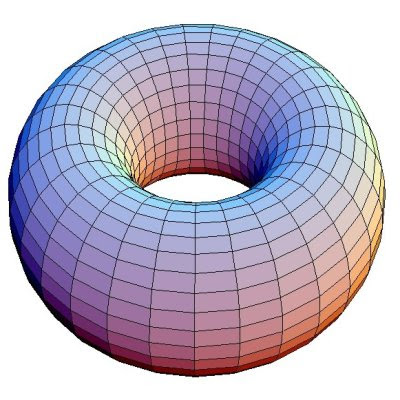However, an examination of the torus in three-space (above) suggests that this is not the case. What is happening here? The answer turns out to be that the folding of the cylinder to the torus distorts the final result, resulting in a manifold with areas of positive and negative curvature. The torus in its true form can only be expressed in four dimensions and higher, and is called a flat torus. Note that the manifold is not truly "flat", but can be constructed in four dimensions from a flat plane without distorting distances.

Similarly, the sphere (fundamental polygon ABB*A*) connects only two of opposite the corners together. The local geometry of a point is less than 360º, and the sphere therefore exhibits spherical geometry, an obvious fact.

The lapse in the accurate representation of 2-manifolds in 3-space is even more pronounced when one considers the fundamental polygon below.This fundamental polygon can also be written ABAB*. When constructed, this fundamental polygon takes the form of a manifold known as the Klein Bottle. The first step in construction is the same as the torus, i.e. connecting the sides marked B and forming a cylinder. However, the A sides are facing in opposite directions and the cylinder must be inverted through itself to construct the manifold. This construction is shown below.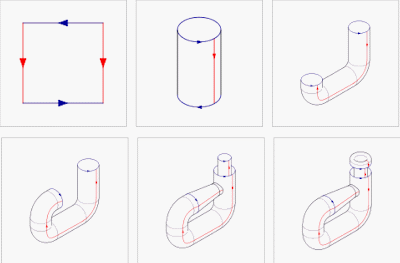The final product is the Klein Bottle.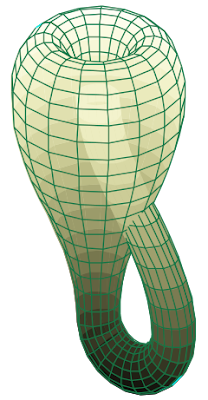It is apparent that self-intersection is necessary in order to construct this manifold in three dimensions. As a result, this too gives only a limited view of its actual structure. The addition of another dimension is needed to eliminate the self-intersection, and to show that this manifold too has Euclidean geometry everywhere.

Yet another fundamental polygon ABAB, produces another manifold that cannot be constructed in three dimensions without self-intersection. It is known as the real projective plane. It has many interesting properties, including its geometric construction. It is the most "difficult" to construct out of the fundamental squares because both pairs of opposite sides are orientated in different directions. Geometrically speaking, it is the set of all lines through the origin in Euclidean 3-space connected into a surface, although this gives little insight to its shape.

More complicated manifolds can be constructed from larger fundamental polygons (all with an even number of sides, of course, because one side must line up with another). For example, consider the hexagon ABCC*B*A* (shown below).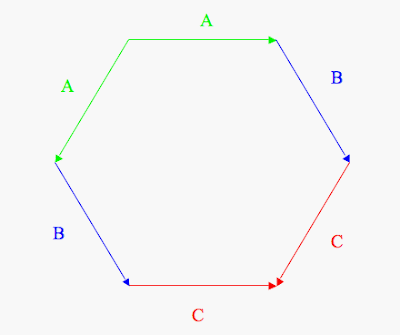This fundamental polygon produces the sphere, just as the ABB*A* does, since only a single diagonal fold is necessary to connect all of the indicated sides. Similarly, ABCB*A*C* produces the torus, ABCB*AC* the Klein Bottle, and ABCBAC, the real projective plane. These are all merely extensions of the fundamental squares, as two sides facing the same direction can be collapsed into one. Take for example the torus, ABCB*A*C*.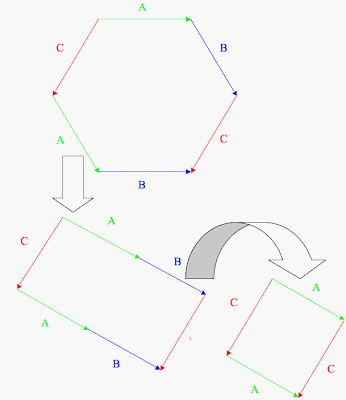Since continuous deformation still preserves the identity of a manifold, the same goes for its fundamental polygon. The above hexagon can therefore be adjusted into a rectangle. The sides A and B are in the same orientation and position relative to each other, they can be combined into a single side while still representing the same idea. The other examples above can be similarly collapsed into fundamental squares.

Fundamental polygon formulas also exist to generate the simplest surface of any given genus, i.e. the n-fold torus. For the two-holed torus, the fundamental polygon is the octagon ABA*B*CDC*D*, which under inspection, is simply two tori fundamental squares "added" together, corresponding to the "gluing" of two tori together to create the double torus. This is equal to ABA*B*+CDC*D*. All eight angles of the polygon coincide where the two tori share a boundary, and the double torus is therefore a hyperbolic manifold. (each angle of a octagon=135º, 8*135º=1080º>360º) With continuous deformation, the point of coincidence can be moved to any point on a double torus, and the manifold therefore has hyperbolic geometry anywhere.

As one adds more tori to the fundamental polygon, the manifold becomes increasingly distorted. It fact, it is "difficult" to draw an n-fold torus with more than three holes in Euclidean 3-space. The general formula is

ABA*B*A'B'A'*B'*A''B''A''*B''*...

which produces an n-fold torus, with each sequence of four letters (ABA*B*, A'B'A'*B'*, A''B''A''*B''*, etc.) represents a single torus that is glued to all others.

As with many aspects of manifolds, the idea of a fundamental polygon may be extended to higher dimensions. For 3-manifolds, fundamental polyhedra take on this role. For example, one might specify a manifold as the resulting figure when opposite faces of a cube are connected. Such connections cannot be visualized in Euclidean 3-space.

Finally, since deformation is permitted, even differently shaped n-faces of any closed polytope of any dimension can be connected to form a manifold. Untold multitudes of manifolds can be produced this way.

The next post of the Manifolds Series deals with the application of manifolds to the shape of the Universe.

Sources: http://en.wikipedia.org/wiki/Fundamental_polygon, The Poincare Conjecture by Donal O'Shea